The KDE Procedure

## Fast Fourier Transform

As shown in the last subsection, kernel density estimates can be expressed as a submatrix of a certain convolution. The fast Fourier transform (FFT) is a computationally effective method for computing such convolutions. For a reference on this material, refer to Press et al. (1988).

The discrete Fourier transform of a complex vector z = (z0, ... ,zN-1) is the vector Z = (Z0, ... ,ZN-1) where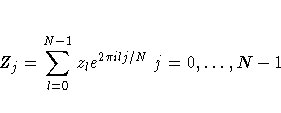and i is the square root of -1. The vector z can be recovered from Z by applying the inverse discrete Fourier transform formula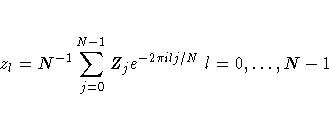Discrete Fourier transforms and their inverses can be computed quickly using the FFT algorithm, especially when N is highly composite; that is, it can be decomposed into many factors, such as a power of 2. By the Discrete Convolution Theorem, the convolution of two vectors is the inverse Fourier transform of the element-by-element product of their Fourier transforms. This, however, requires certain periodicity assumptions, which explains why the vectors K and C require zero-padding. This is to avoid "wrap-around" effects (refer to Press et al. 1988, pp. 410 - 411). The vector K is actually mirror-imaged so that the convolution of C and K will be the vector of binned estimates. Thus, if S denotes the inverse Fourier transform of the element-by-element product of the Fourier transforms of K and C, then the first g elements of S are the estimates.

The bivariate Fourier transform of an N1×N2 complex matrix having (l1+1,l2+1) entry equal to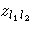is the N1×N2 matrix with (j1+1 ,j2+1) entry given by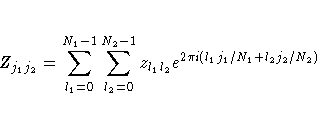and the formula of the inverse is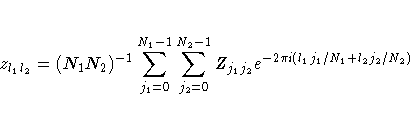The same Discrete Convolution Theorem applies, and zero-padding is needed for matrices C and K. In the case of K, the matrix is mirror-imaged twice. Thus, if S denotes the inverse Fourier transform of the element-by-element product of the Fourier transforms of K and C, then the upper-left gX×gY corner of S contains the estimates.